# ML Aggarwal Solutions for Class 8 Maths Chapter 15 Circle

ML Aggarwal Solutions for Class 8 Maths Chapter 15 Circle has accurate answers designed by a set of expert faculty at BYJU’S. Students who want to excel in exams, are suggested to follow ML Aggarwal Solutions as a reference guide. Practising these solutions helps them to understand the complex concepts effortlessly. This also enhances analytical and logical thinking abilities among students, which are important from the exam perspective. To speed up the problem solving skills, ML Aggarwal Solutions for Class 8 Maths Chapter 15 Circle PDF, links are available with a free download option.

Chapter 15 explains all the fundamental concepts related to Circle. Construction of the Circle is the main concept discussed here. Circle is one of the significant chapters, as it continues in higher classes as well. Students can analyse their exam preparation by cross checking their answers while solving textbook problems.

## ML Aggarwal Solutions for Class 8 Maths Chapter 15 Circle Download PDF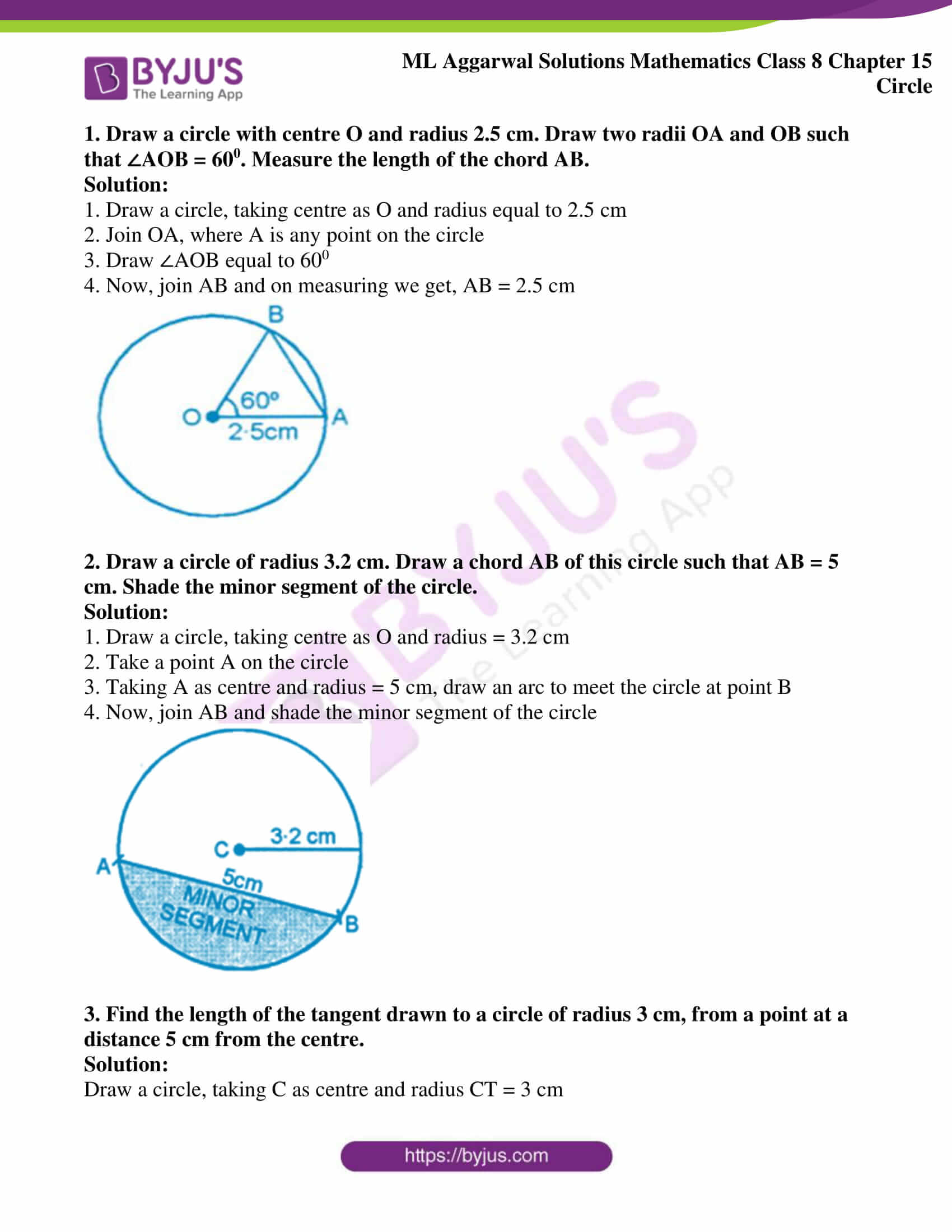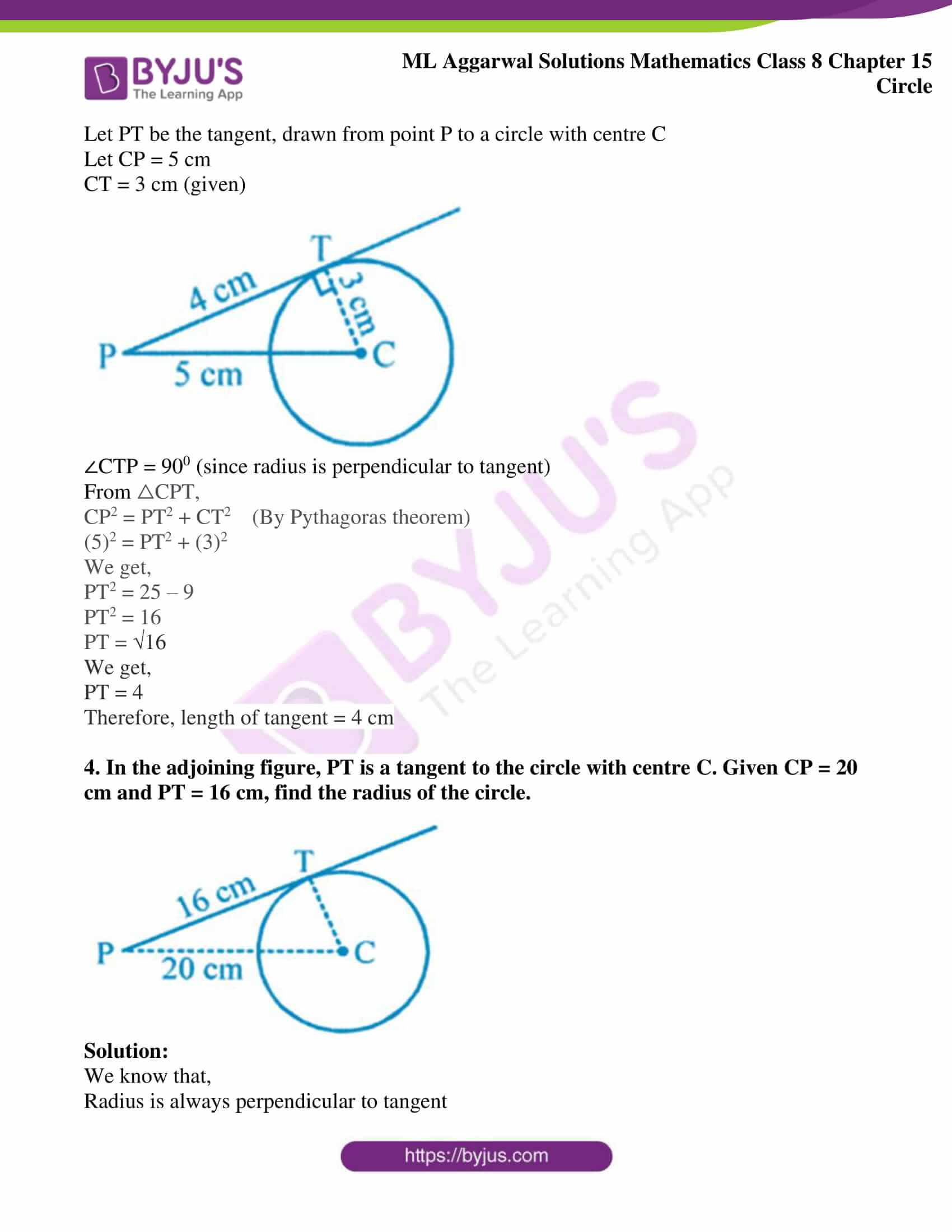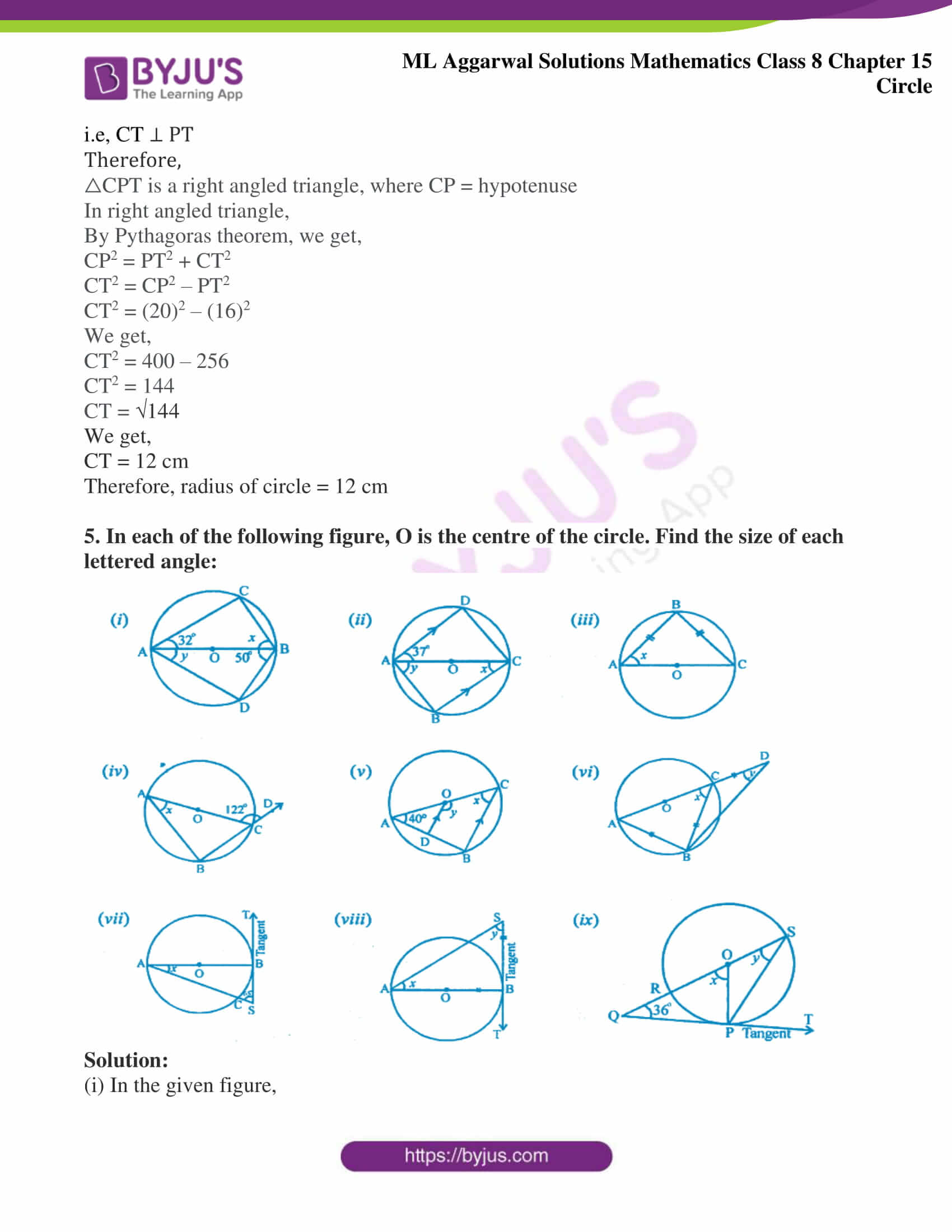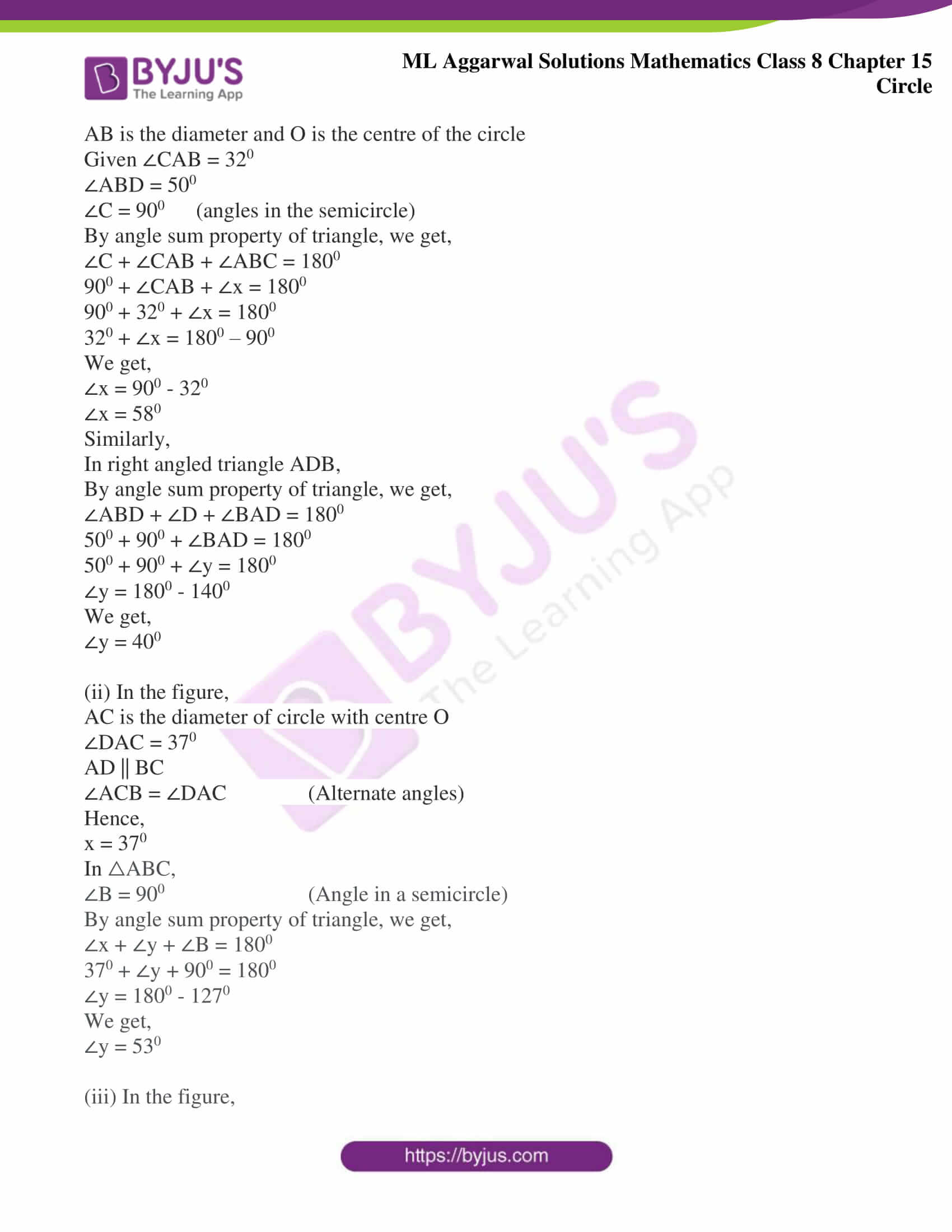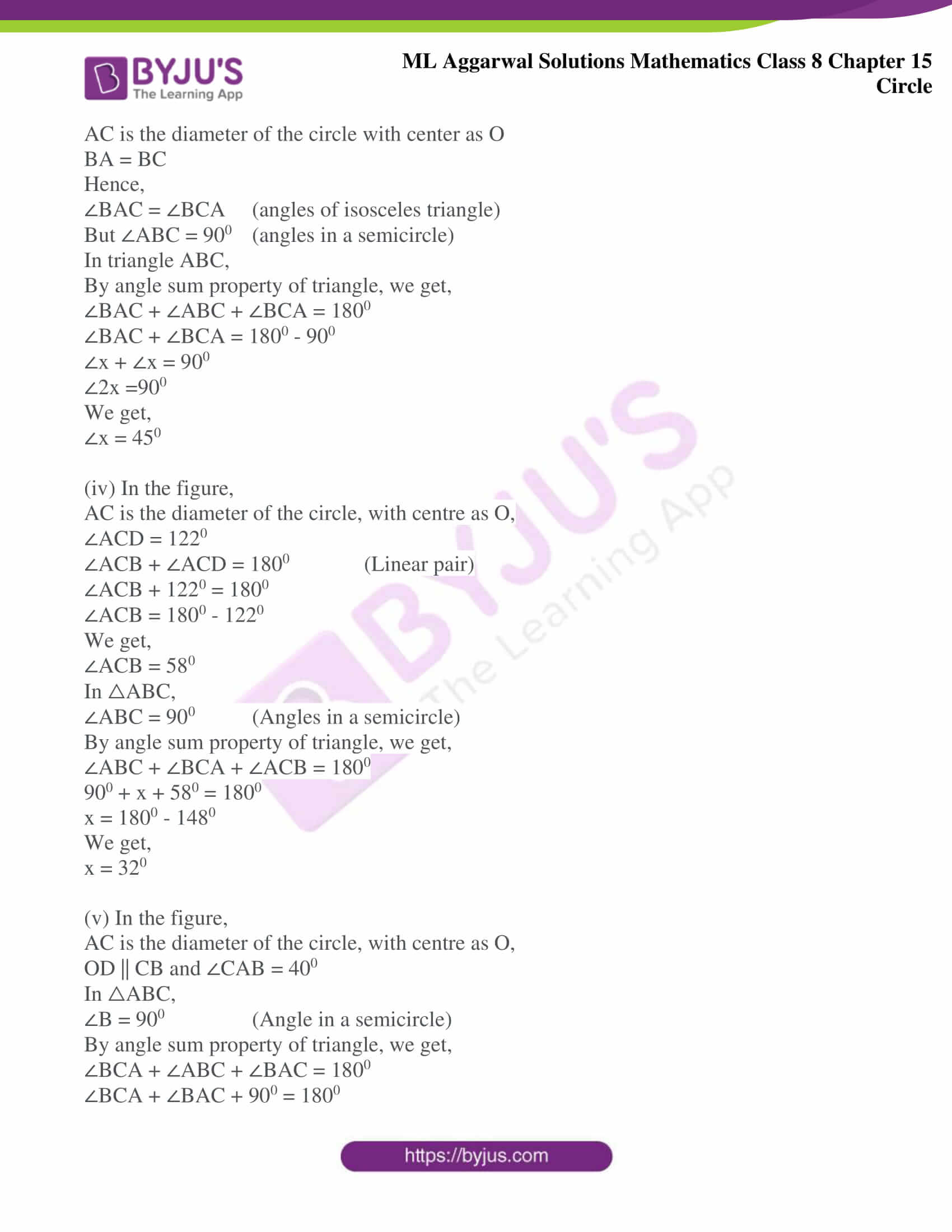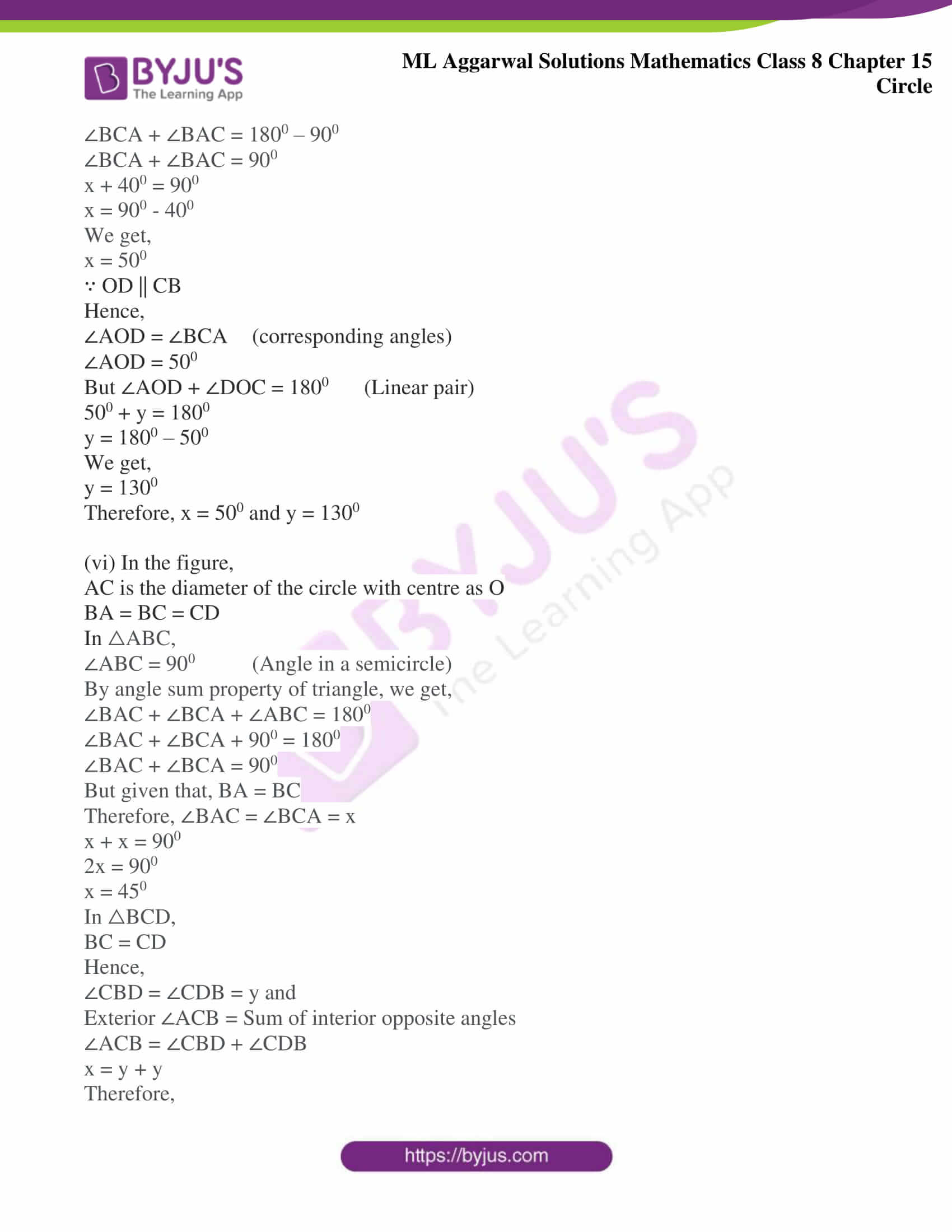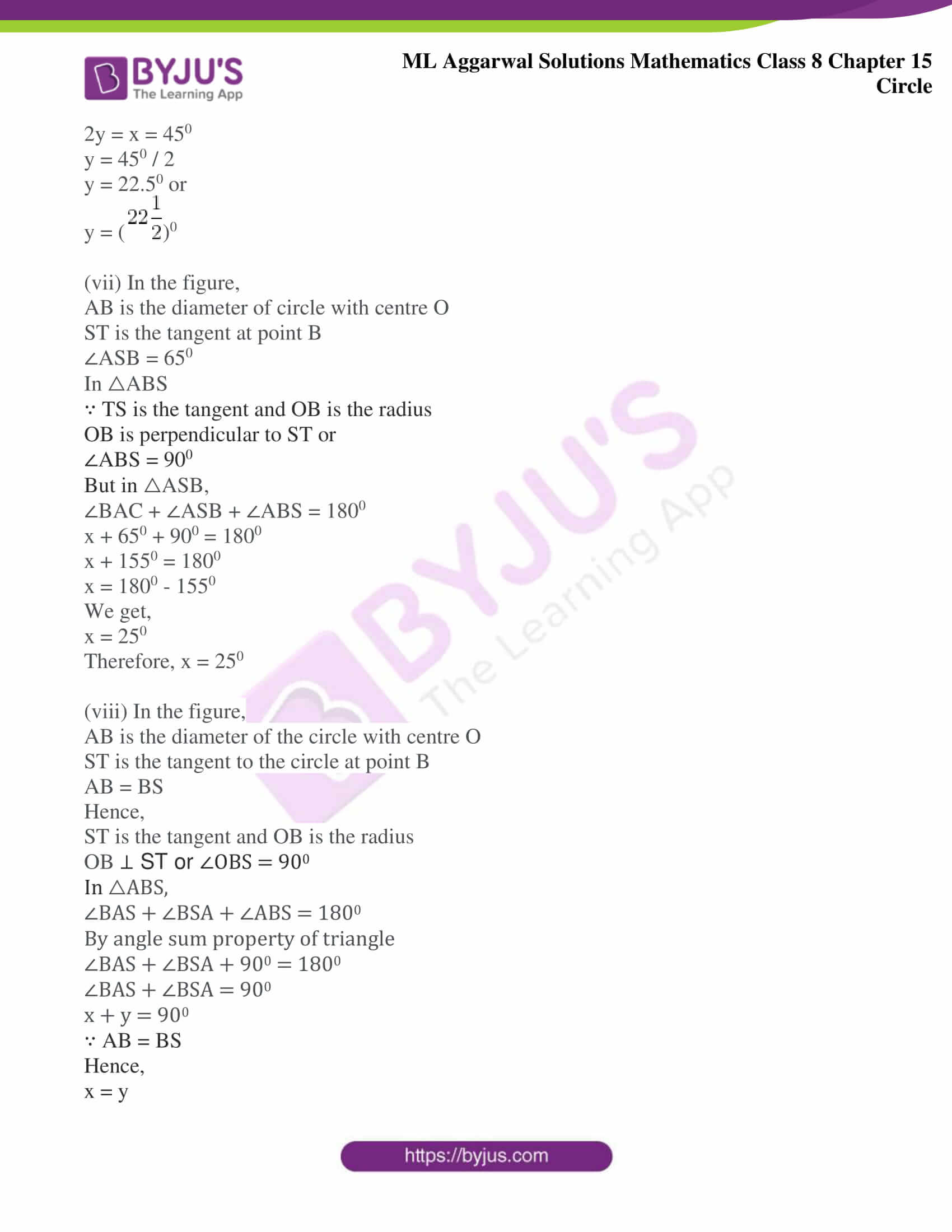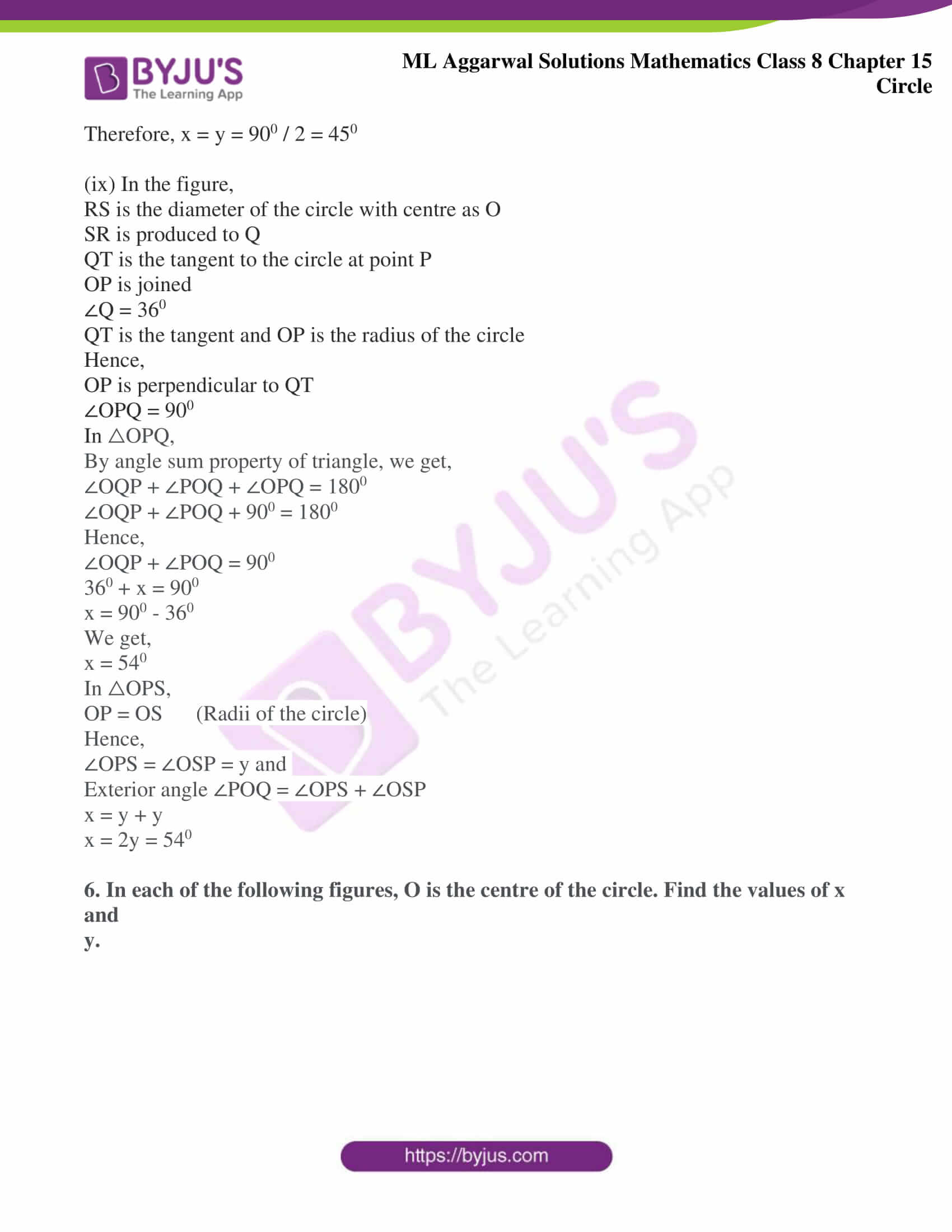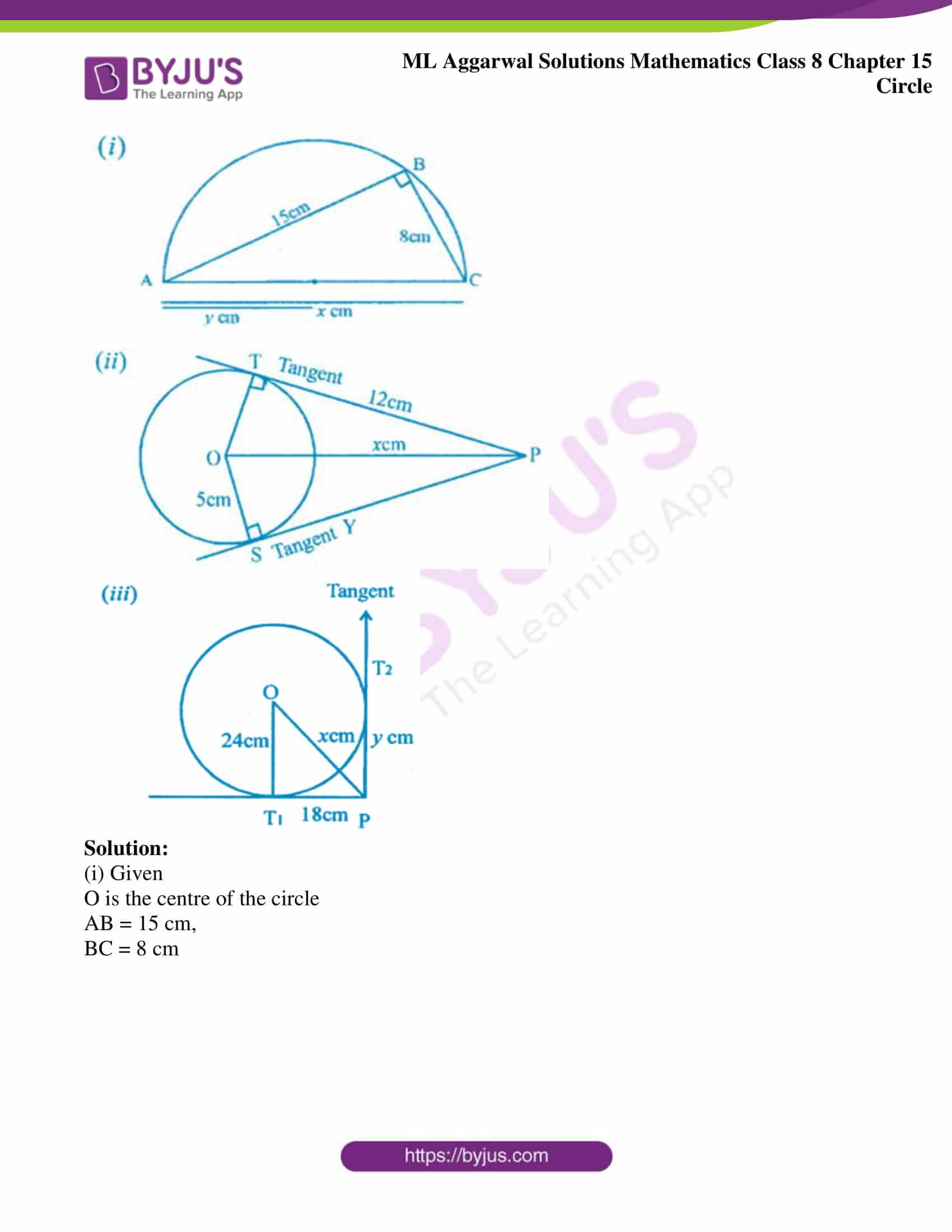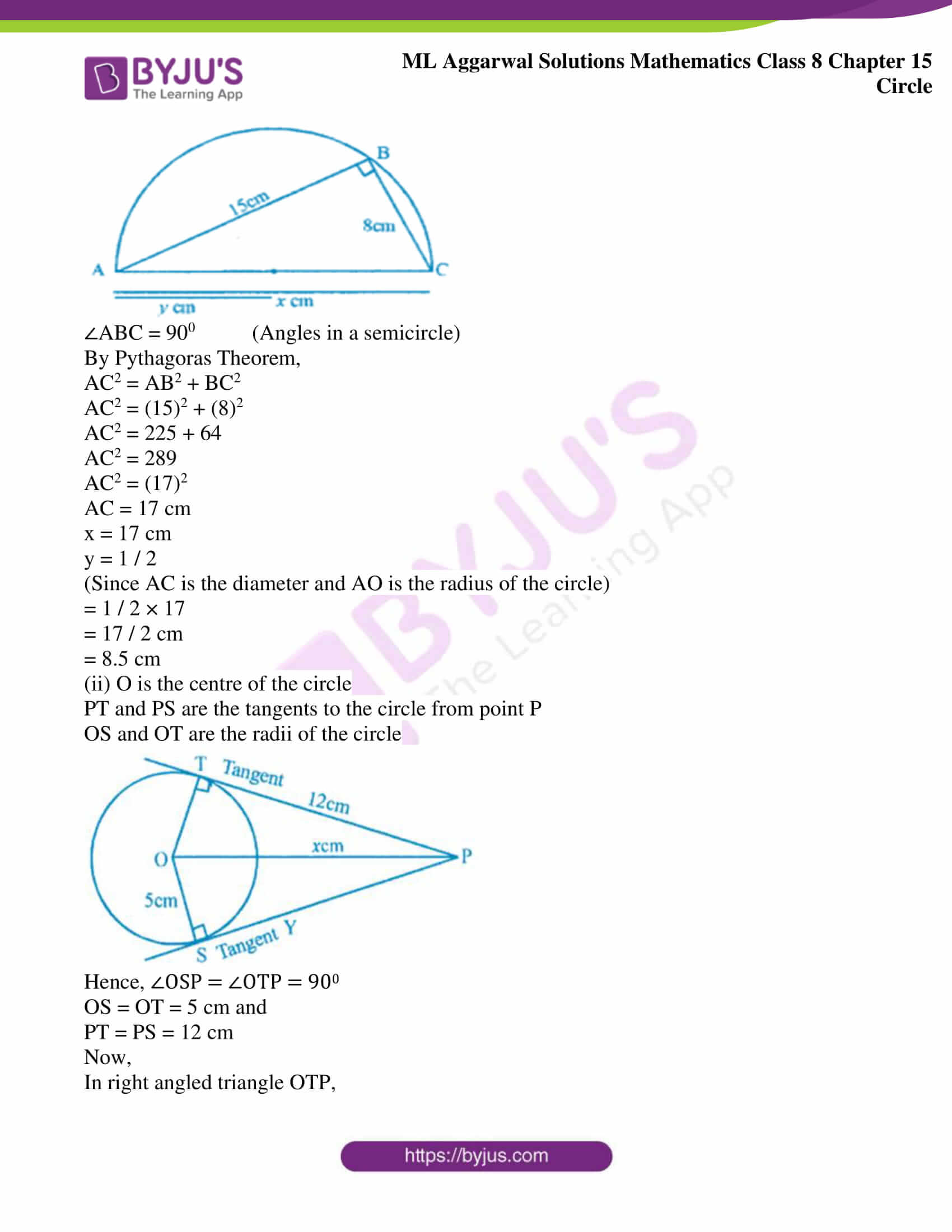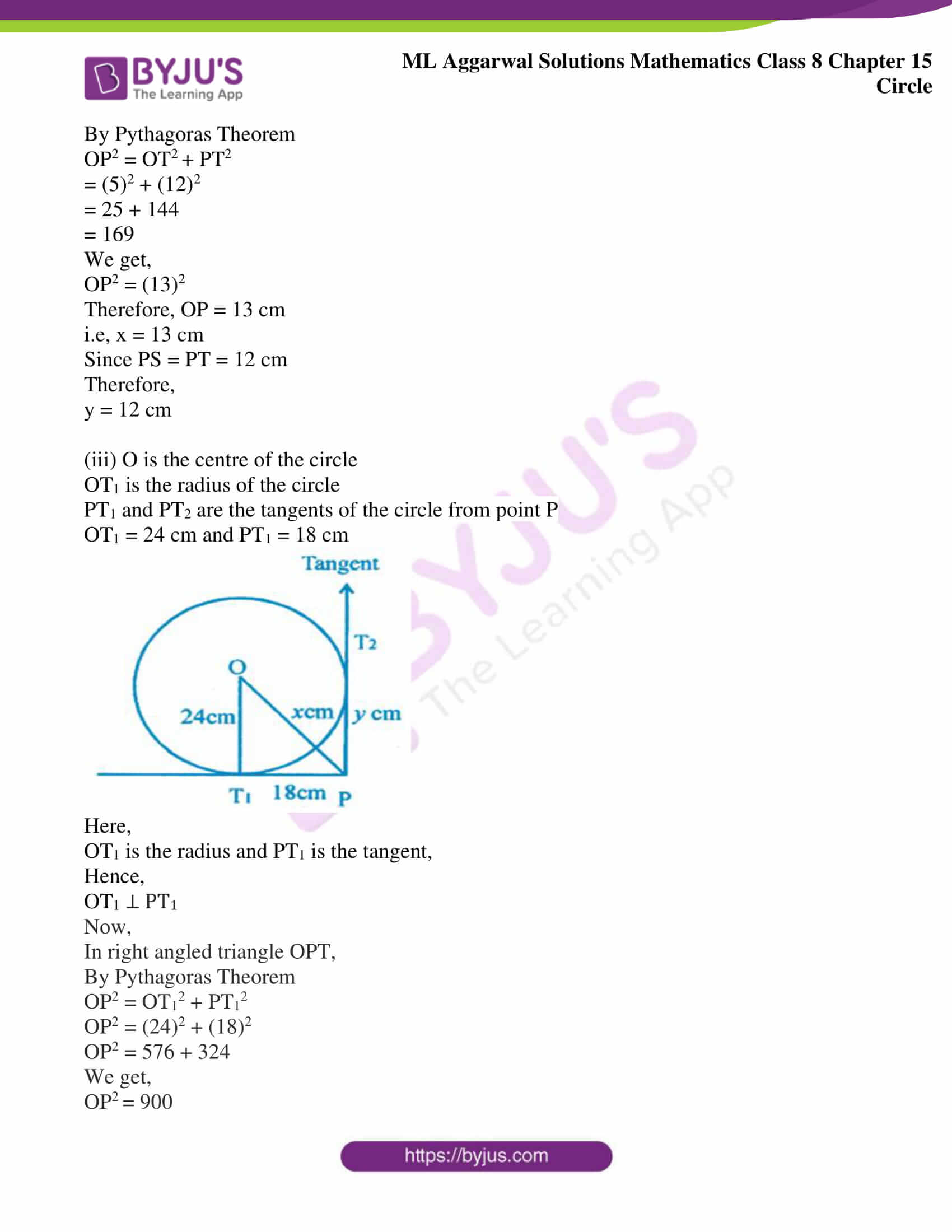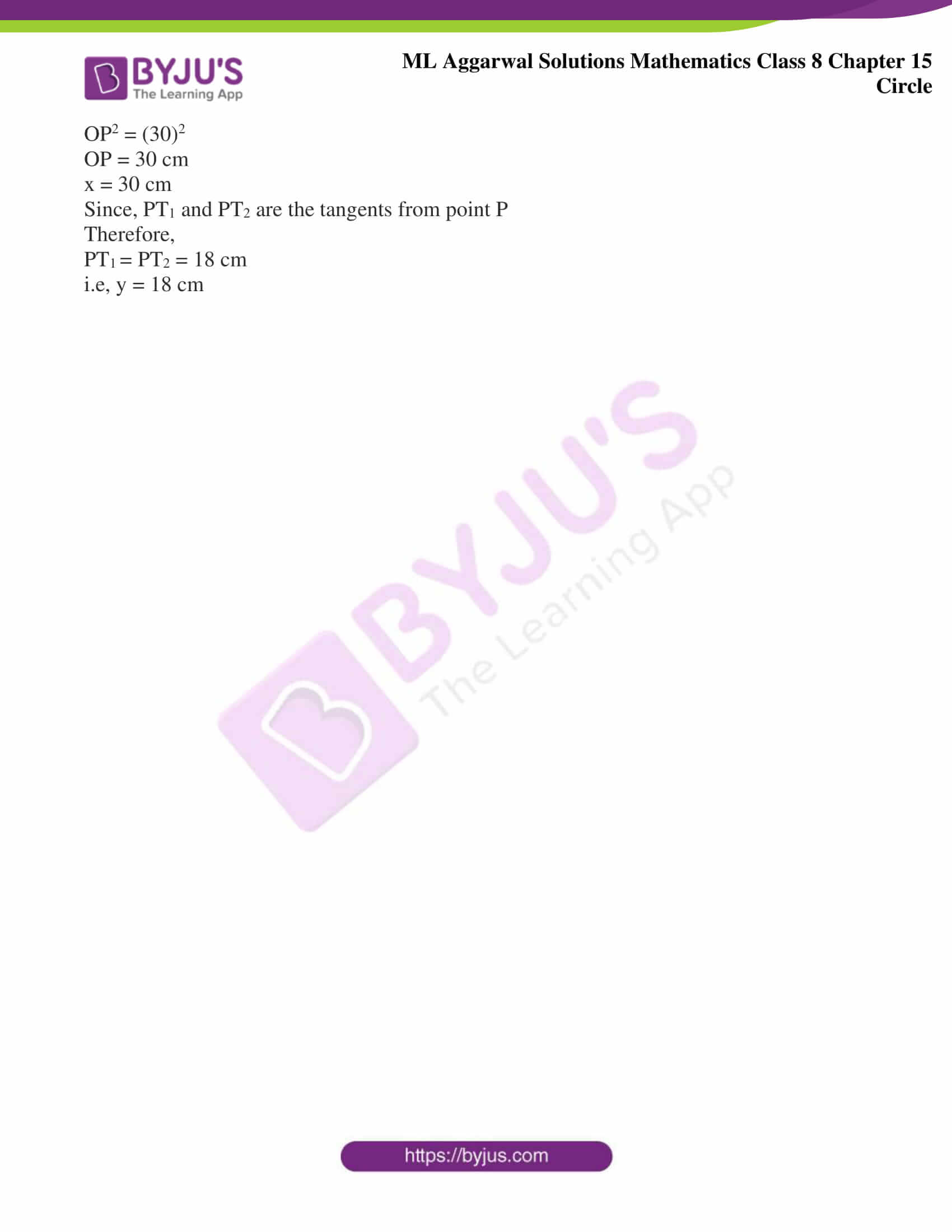## Access ML Aggarwal Solutions for Class 8 Maths Chapter 15 Circle

1. Draw a circle with centre O and radius 2.5 cm. Draw two radii OA and OB such that ∠AOB = 600. Measure the length of the chord AB.

Solution:

1. Draw a circle, taking centre as O and radius equal to 2.5 cm

2. Join OA, where A is any point on the circle

3. Draw ∠AOB equal to 600

4. Now, join AB and on measuring we get, AB = 2.5 cm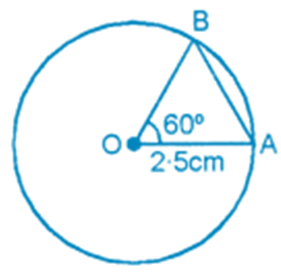2. Draw a circle of radius 3.2 cm. Draw a chord AB of this circle such that AB = 5 cm. Shade the minor segment of the circle.

Solution:

1. Draw a circle, taking centre as O and radius = 3.2 cm

2. Take a point A on the circle

3. Taking A as centre and radius = 5 cm, draw an arc to meet the circle at point B

4. Now, join AB and shade the minor segment of the circle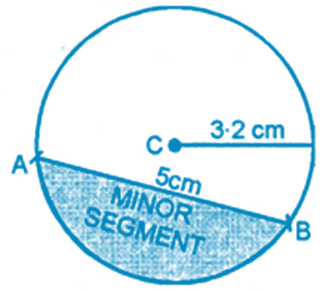3. Find the length of the tangent drawn to a circle of radius 3 cm, from a point at a distance 5 cm from the centre.

Solution:

Draw a circle, taking C as centre and radius CT = 3 cm

Let PT be the tangent, drawn from point P to a circle with centre C

Let CP = 5 cm

CT = 3 cm (given)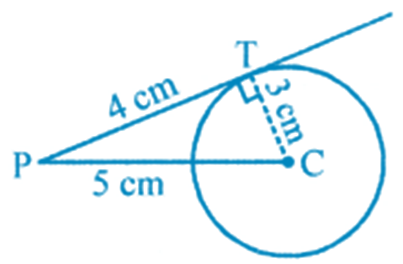∠CTP = 900 (since radius is perpendicular to tangent)

From △CPT,

CP2 = PT2 + CT2 (By Pythagoras theorem)

(5)2 = PT2 + (3)2

We get,

PT2 = 25 – 9

PT2 = 16

PT = 16

We get,

PT = 4

Therefore, length of tangent = 4 cm

4. In the adjoining figure, PT is a tangent to the circle with centre C. Given CP = 20 cm and PT = 16 cm, find the radius of the circle.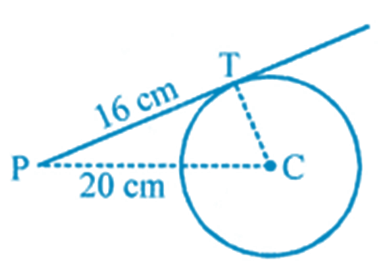Solution:

We know that,

Radius is always perpendicular to tangent

i.e, CT ⊥ PT

Therefore,

△CPT is a right angled triangle, where CP = hypotenuse

In right angled triangle,

By Pythagoras theorem, we get,

CP2 = PT2 + CT2

CT2 = CP2 – PT2

CT2 = (20)2 – (16)2

We get,

CT2 = 400 – 256

CT2 = 144

CT = 144

We get,

CT = 12 cm

Therefore, radius of circle = 12 cm

5. In each of the following figure, O is the centre of the circle. Find the size of each lettered angle: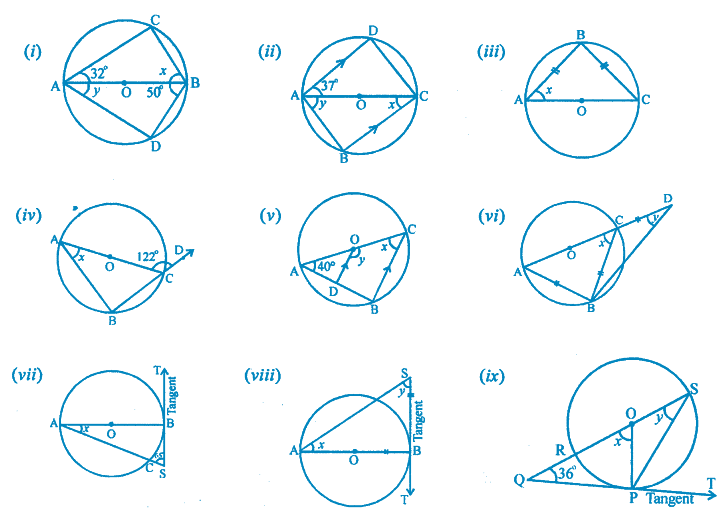Solution:

(i) In the given figure,

AB is the diameter and O is the centre of the circle

Given ∠CAB = 320

∠ABD = 500

∠C = 900 (angles in the semicircle)

By angle sum property of triangle, we get,

∠C + ∠CAB + ∠ABC = 1800

900 + ∠CAB + ∠x = 1800

900 + 320 + ∠x = 1800

320 + ∠x = 1800 – 900

We get,

∠x = 900 – 320

∠x = 580

Similarly,

By angle sum property of triangle, we get,

∠ABD + ∠D + ∠BAD = 1800

500 + 900 + ∠BAD = 1800

500 + 900 + ∠y = 1800

∠y = 1800 – 1400

We get,

∠y = 400

(ii) In the figure,

AC is the diameter of circle with centre O

∠DAC = 370

∠ACB = ∠DAC (Alternate angles)

Hence,

x = 370

In △ABC,

∠B = 900 (Angle in a semicircle)

By angle sum property of triangle, we get,

∠x + ∠y + ∠B = 1800

370 + ∠y + 900 = 1800

∠y = 1800 – 1270

We get,

∠y = 530

(iii) In the figure,

AC is the diameter of the circle with center as O

BA = BC

Hence,

∠BAC = ∠BCA (angles of isosceles triangle)

But ∠ABC = 900 (angles in a semicircle)

In triangle ABC,

By angle sum property of triangle, we get,

∠BAC + ∠ABC + ∠BCA = 1800

∠BAC + ∠BCA = 1800 – 900

∠x + ∠x = 900

∠2x =900

We get,

∠x = 450

(iv) In the figure,

AC is the diameter of the circle, with centre as O,

∠ACD = 1220

∠ACB + ∠ACD = 1800 (Linear pair)

∠ACB + 1220 = 1800

∠ACB = 1800 – 1220

We get,

∠ACB = 580

In △ABC,

∠ABC = 900 (Angles in a semicircle)

By angle sum property of triangle, we get,

∠ABC + ∠BCA + ∠ACB = 1800

900 + x + 580 = 1800

x = 1800 – 1480

We get,

x = 320

(v) In the figure,

AC is the diameter of the circle, with centre as O,

OD || CB and ∠CAB = 400

In △ABC,

∠B = 900 (Angle in a semicircle)

By angle sum property of triangle, we get,

∠BCA + ∠ABC + ∠BAC = 1800

∠BCA + ∠BAC + 900 = 1800

∠BCA + ∠BAC = 1800 – 900

∠BCA + ∠BAC = 900

x + 400 = 900

x = 900 – 400

We get,

x = 500

∵ OD || CB

Hence,

∠AOD = ∠BCA (corresponding angles)

∠AOD = 500

But ∠AOD + ∠DOC = 1800 (Linear pair)

500 + y = 1800

y = 1800 – 500

We get,

y = 1300

Therefore, x = 500 and y = 1300

(vi) In the figure,

AC is the diameter of the circle with centre as O

BA = BC = CD

In △ABC,

∠ABC = 900 (Angle in a semicircle)

By angle sum property of triangle, we get,

∠BAC + ∠BCA + ∠ABC = 1800

∠BAC + ∠BCA + 900 = 1800

∠BAC + ∠BCA = 900

But given that, BA = BC

Therefore, ∠BAC = ∠BCA = x

x + x = 900

2x = 900

x = 450

In △BCD,

BC = CD

Hence,

∠CBD = ∠CDB = y and

Exterior ∠ACB = Sum of interior opposite angles

∠ACB = ∠CBD + ∠CDB

x = y + y

Therefore,

2y = x = 450

y = 450 / 2

y = 22.50 or

y = (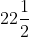)0

(vii) In the figure,

AB is the diameter of circle with centre O

ST is the tangent at point B

∠ASB = 650

In △ABS

∵ TS is the tangent and OB is the radius

OB is perpendicular to ST or

∠ABS = 900

But in △ASB,

∠BAC + ∠ASB + ∠ABS = 1800

x + 650 + 900 = 1800

x + 1550 = 1800

x = 1800 – 1550

We get,

x = 250

Therefore, x = 250

(viii) In the figure,

AB is the diameter of the circle with centre O

ST is the tangent to the circle at point B

AB = BS

Hence,

ST is the tangent and OB is the radius

OB ⊥ ST or ∠OBS = 900

In △ABS,

∠BAS + ∠BSA + ∠ABS = 1800

By angle sum property of triangle

∠BAS + ∠BSA + 900 = 1800

∠BAS + ∠BSA = 900

x + y = 900

∵ AB = BS

Hence,

x = y

Therefore, x = y = 900 / 2 = 450

(ix) In the figure,

RS is the diameter of the circle with centre as O

SR is produced to Q

QT is the tangent to the circle at point P

OP is joined

∠Q = 360

QT is the tangent and OP is the radius of the circle

Hence,

OP is perpendicular to QT

∠OPQ = 900

In △OPQ,

By angle sum property of triangle, we get,

∠OQP + ∠POQ + ∠OPQ = 1800

∠OQP + ∠POQ + 900 = 1800

Hence,

∠OQP + ∠POQ = 900

360 + x = 900

x = 900 – 360

We get,

x = 540

In △OPS,

OP = OS (Radii of the circle)

Hence,

∠OPS = ∠OSP = y and

Exterior angle ∠POQ = ∠OPS + ∠OSP

x = y + y

x = 2y = 540

6. In each of the following figures, O is the centre of the circle. Find the values of x and

y.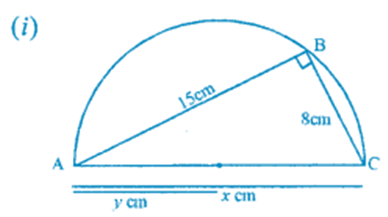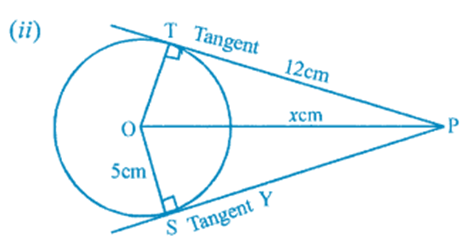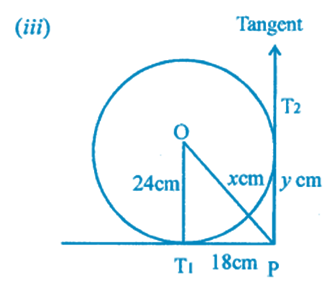Solution:

(i) Given

O is the centre of the circle

AB = 15 cm,

BC = 8 cm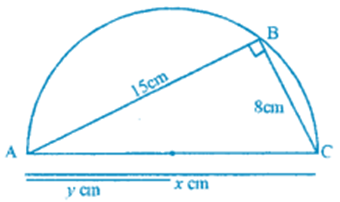∠ABC = 900 (Angles in a semicircle)

By Pythagoras Theorem,

AC2 = AB2 + BC2

AC2 = (15)2 + (8)2

AC2 = 225 + 64

AC2 = 289

AC2 = (17)2

AC = 17 cm

x = 17 cm

y = 1 / 2

(Since AC is the diameter and AO is the radius of the circle)

= 1 / 2 × 17

= 17 / 2 cm

= 8.5 cm

(ii) O is the centre of the circle

PT and PS are the tangents to the circle from point P

OS and OT are the radii of the circle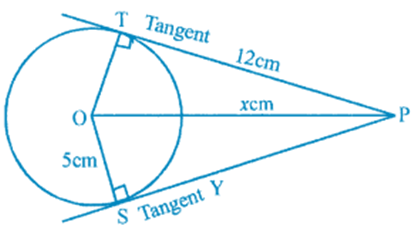Hence, ∠OSP = ∠OTP = 900

OS = OT = 5 cm and

PT = PS = 12 cm

Now,

In right angled triangle OTP,

By Pythagoras Theorem

OP2 = OT2 + PT2

= (5)2 + (12)2

= 25 + 144

= 169

We get,

OP2 = (13)2

Therefore, OP = 13 cm

i.e, x = 13 cm

Since PS = PT = 12 cm

Therefore,

y = 12 cm

(iii) O is the centre of the circle

OT1 is the radius of the circle

PT1 and PT2 are the tangents of the circle from point P

OT1 = 24 cm and PT1 = 18 cm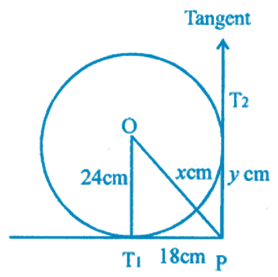Here,

OT1 is the radius and PT1 is the tangent,

Hence,

OT1 ⊥ PT1

Now,

In right angled triangle OPT,

By Pythagoras Theorem

OP2 = OT12 + PT12

OP2 = (24)2 + (18)2

OP2 = 576 + 324

We get,

OP2 = 900

OP2 = (30)2

OP = 30 cm

x = 30 cm

Since, PT1 and PT2 are the tangents from point P

Therefore,

PT1 = PT2 = 18 cm

i.e, y = 18 cm